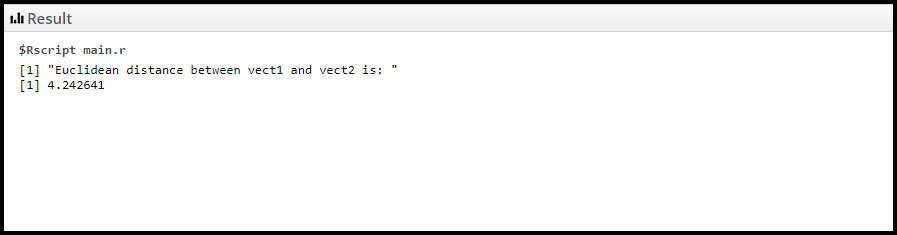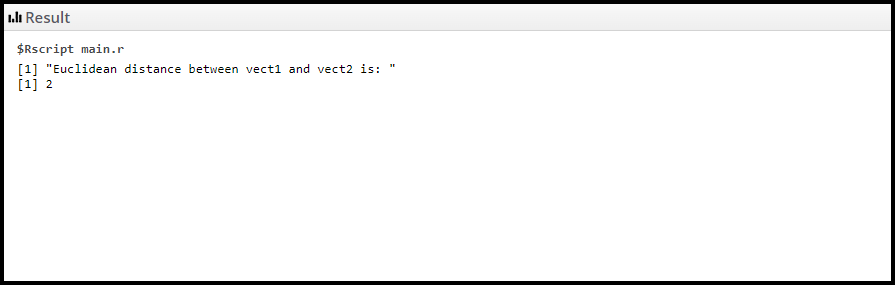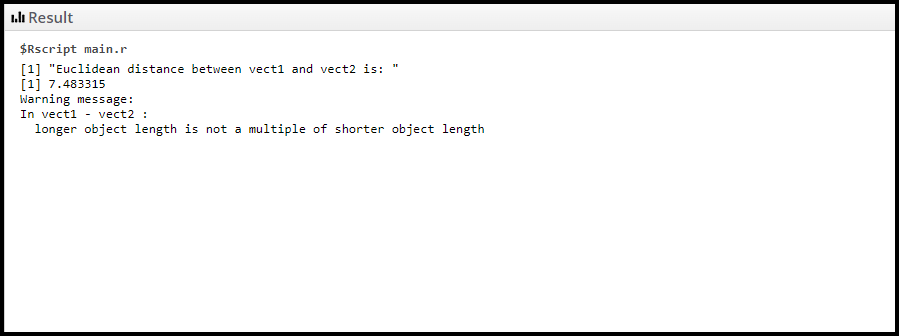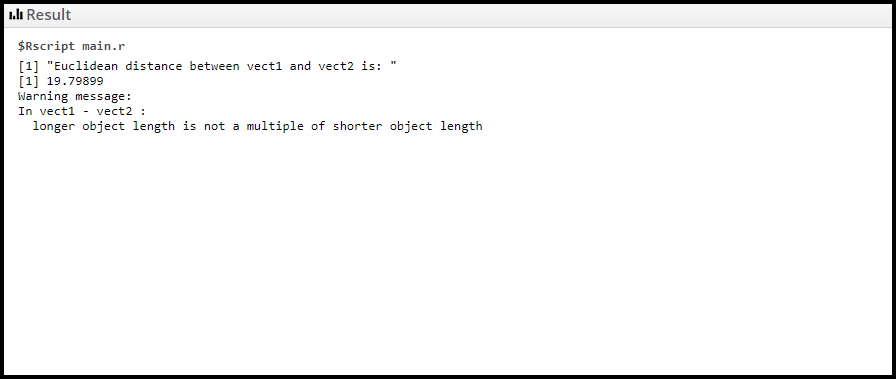# How to Calculate Euclidean Distance in R?

• Last Updated : 28 Nov, 2021

Euclidean distance between two points in Euclidean space is the length of a line segment between the two points. It can be calculated from the Cartesian coordinates of the points using the Pythagorean theorem, therefore occasionally being called the Pythagorean distance. The Euclidean distance between the two vectors is given by

`√Σ(vect1i - vect2i)2`

where,

• vect1 is the first vector
• vect2 is the second vector

For example, we are given two vectors, vect1 as (1, 4, 3, 5) and vect2 as (2, 3, 2, 4). Their Euclidean distance is given by, √(1 – 2)2 + (4 – 3)2 + (3 – 2)2 + (5 – 4)2   which is equal to 2. Below is the implementation using two vectors of equal length:

Example 1:

## R

 `# Function to calculate Euclidean distance``# Sum function calculates the sum of the ``# squares of absolute difference  between``# corresponding elements of vect1 and vect2``CalculateEuclideanDistance <- ``function``(vect1, vect2) ``sqrt``(``sum``((vect1 - vect2)^2))`` ` `# Initializing two vectors having equal length``vect1 <- ``c``(2, 4, 4, 7)``vect2 <- ``c``(1, 2, 2, 10)`` ` `print``(``"Euclidean distance between vect1 and vect2 is: "``)`` ` `# Calling CalculateEuclideanDistance function ``CalculateEuclideanDistance``(vect1, vect2)`

Output:Example 2:

## R

 `# Function to calculate Euclidean distance``# Sum function calculates the sum of the ``# squares of absolute difference between``# corresponding elements of vect1 and vect2``CalculateEuclideanDistance <- ``function``(vect1, vect2) ``sqrt``(``sum``((vect1 - vect2)^2))`` ` `# Initializing two vectors having equal length``vect1 <- ``c``(2, 3, 4, 7)``vect2 <- ``c``(1, 2, 3, 8)`` ` `print``(``"Euclidean distance between vect1 and vect2 is: "``)`` ` `# Calling CalculateEuclideanDistance function``CalculateEuclideanDistance``(vect1, vect2)`

Output:If the two vectors have unequal length then the compiler gives a warning message. Below is the implementation using two vectors having unequal length.

Example 3:

## R

 `# Function to calculate Euclidean distance``# Sum function calculates the sum of the ``# squares of absolute difference between``# corresponding elements of vect1 and vect2``CalculateEuclideanDistance <- ``function``(vect1, vect2) ``sqrt``(``sum``((vect1 - vect2)^2))`` ` `# Initializing two vectors having equal length``vect1 <- ``c``(4, 3, 4, 8)``vect2 <- ``c``(3, 2, 3, 1, 2)`` ` `print``(``"Euclidean distance between vect1 and vect2 is: "``)`` ` `# Calling CalculateEuclideanDistance function ``CalculateEuclideanDistance``(vect1, vect2)`

Output:As you can see in the output, the compiler gives us a warning since the length of vect1 is shorter than vect2.

Example 4:

## R

 `# Function to calculate Euclidean distance``# Sum function calculates the sum of the``# squares of absolute difference between``# corresponding elements of vect1 and vect2``CalculateEuclideanDistance <- ``function``(vect1, vect2) ``sqrt``(``sum``((vect1 - vect2)^2))`` ` `# Initializing two vectors having equal length``vect1 <- ``c``(1, 7, 1, 3, 10, 15 )``vect2 <- ``c``(3, 2, 10, 11 )`` ` `print``(``"Euclidean distance between vect1 and vect2 is: "``)`` ` `# Calling CalculateEuclideanDistance function ``CalculateEuclideanDistance``(vect1, vect2)`

Output:As you can see in the output, the compiler gives us a warning since the length of vect2 is shorter than vect1.

My Personal Notes arrow_drop_up